Illustrations

f:fractions

# Fractions

A fraction is part of a unit, and is written with two figures, one above the other, with a line between them: thus,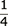,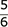,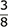. The upper figure is called the numerator, and the lower figure the denominator. The denominator shows how many parts the unit is divided into; and the numerator, how many of those parts the fraction represents. A proper fraction is when the numerator is less than the denominator. An improper fraction is when the numerator is equal to, or greater than the denominator.

A compound fraction is the fraction of a fraction, and is known by the word, of, between the parts; as,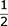of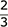of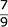of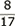. A mixed number is composed of a whole number and fraction; as,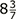,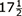. A mixed fraction

is when the numerator or denominator has a fractional part; thus,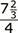,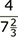; or, when both of them have fractional parts; as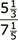.

The fractions cast in one piece to a fount are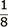,,,,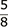,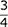,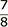. Fractions are also cast in two pieces, from Brevier to English; when wanted larger, they may be formed of figures of small proportionate size, with a rule between the numerator and denominator.

This rule in separate fractions is now generally cast on the piece containing the denominator, above the figure, thus, 1 2 3 4 5 6 7 8 9 0; but in improper fractions, where the numerator contains more figures than the denominator, as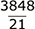this rule will not cover the whole of the numerator, therefore it would be necessary, to be able to compose fractions in a perfect manner, to have the rule also cast under the numerator, according to the old plan, so as to meet both cases, otherwise either the proper fraction or the improper fraction must be deficient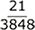.

### Page Tools